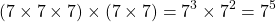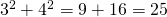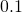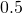# Integer Exponents

Let’s examine, where(integers).

We define the exponent to be a short hand notation for repeated multiplication.

A summary of the laws can be found here.

# The Product Law

We know that. Let’s write that in another way:orNotice thatand that.

Let’s take a second example: suppose we have.

In full:.

This leads us to the exponent law:To generalise further:# The Quotient Law

Remember that a fraction is a way to write division. For example:We also know that if a fraction has a common factor on numerator and denominator, it can be simplified. For example:Usually, we don’t write out all of those steps. You might be more familiar with ‘scoring out’ or ‘cancelling’. Showing the steps without scoring out shows why scoring out works mathematically.

Let’s see how simplifying fractions helps with exponents. This time, let’s use ‘scoring out’.Or, in other words, we see a common factor ofon the numerator and the denominator. How many 7’s did we ‘score out’? We started with 5, we scored out 3, and we were left with 2. This leads us to the law for the quotient of two powers with the same base:or, more generally,# Negative Exponents

The quotient law leads us to negative indices. Suppose we have.In summary,Or, in generalWhen we saywe mean,. When we saywe mean one divided bythree times over, that is:which is really awkward notation and is not often used. Better to say:On the applet below, move the slider to the right to multiply by, move to the left to divide by. Let the ‘initial value’ be. A positive exponent returns the number of times we multiplyby. A negative exponent returns the number of times we divideby. The exponentmeans that we have neither multiplied nor divided the numberby.

# Exponent Zero

Now suppose we have. Well, we already know that anything divided by itself is just 1.

Let’s see what happens when we apply the quotient law:In general,(with the curious exception of a=0, but only sometimes…).

# The Power Law

The power law tells us what do when we have a power of a power:In general,.

# Distribution over a Product or Quotient

Now suppose we have the number, we know the answer is 100. Let’s write the number 10 as a product and then square it:In other words,This example illustrates that, in general:Similarly, with division:In general,Take Care though – an exponent does not distribute over addition/subtraction!

Example:The common mistake would be to say thatis equal tobut lets figure that out:which is not 49, so that is simply wrong.

# Summary

Click to open the summary!

# Non-Integer Exponents

The explanations on this page assume that the exponents are integers, however we can establish meaning to rational and irrational exponents.

What might the value ofbe? What meaning does it have?

Using the product law, what is the value of?

What about? Does it have any meaning?

See if your calculator can make sense ofand.

By establishing meaning to all real exponents, we are able to define an exponential function such as. This kind of function can be used to model all kinds of exponential growth (or decay) – from population growth (Biology) to compound interest (Finance).

The functionis shown below. Click on the red point to see points plotted at integer values of. Move the red point to thevalueto see that. Click on the green point to seeincrease by. Move the green point to thevaluewe see that the value given to.